Vallorie J. Peridier

## FacetSurface RepresentationNBCDFPDF

### Rough-Surface Simulation and the Visualization of Nonsmooth Arrays of Data

This article considers both the creation of random-looking irregular surfaces and the triangular-facet visualization and interpolation of nonsmooth data arrays. This rough-surface simulation scheme is novel because cellular automata computations define the surface topology. The companion triangular-facet surface-plotting method produces a crisp visualization of nonsmooth data arrays.

### Introduction

This article describes both the computation and the visualization of irregular rough surfaces.

The rough-surface generation scheme is novel because it uses cellular automaton methods to define the surface topology. Users of Mathematica, with its built-in cellular automaton functionality, can employ the algorithm described in this article as a convenient way of devising rough surfaces by manipulating parameters such as the rule number, number of steps, and initial conditions.

The visualization of nonsmooth data arrays is the second topic considered. A data-interpolation facility, consistent with this alternating-diagonal triangular-facet visualization of the data array, is also described.

Both capabilities, rough-surface generation and irregular-data visualization and interpolation, can be used independently or together; both are implemented in the package FacetSurface.m. It is available from content.wolfram.com/uploads/sites/19/2011/07/FacetSurface.m.

### Rough Surfaces Generated by Cellular Automata

#### Background

##### Current Practice for Numerical Simulation of Rough Surfaces

Before describing this new method for simulating rough surfaces with cellular automata, it is worthwhile to note the principal alternative approaches. The rather broad topic of rough-surface simulation is fundamental in studies of wear and friction; two categories of techniques have evolved in this literature. The first, motivated by the early analytical studies of friction , entails the use of probabilistic, statistical, or Monte Carlo methods to distribute characteristic values over a surface (peak height, peak radius of curvature, etc.) [2, 3]. The second and more recent category employs fractal concepts [4, 5]. There are also hybrid approaches that use both statistical and fractal algorithms in tandem [6, 7].

Both the statistical surface-simulation methods and the fractal approaches have a substantial body of literature and their own respective advantages. Probabilistic schemes are physically intuitive and represent surfaces in a manner consistent with the classical analytical treatments of friction . However, fractal methods seem to be more in vogue now, owing in part to the current practice of characterizing empirically studied surfaces using a fractal dimension . Note that surfaces simulated by fractals have a somewhat homogeneous quality that may or may not resemble their physical counterpart with the same reported fractal dimension .

In summary, the two prevailing methodologies for simulating irregular surfaces reflect the modeling traditions and empirical practices of the wear-and-friction literature.

##### This New Cellular Automaton Approach

This new cellular automaton method for simulating rough surfaces was first conceived during the author’s participation at the NKS 2008 Summer School. The method is a departure from the two aforementioned approaches for simulating irregular surfaces: it is neither a fractal method nor a probabilistic scheme and it is not envisaged as a contribution to the literature of wear and friction.

This cellular automaton scheme for simulating rough surfaces is simple in concept: a grid of numbers, generated by accumulating a two-dimensional cellular automaton evolution, is used to define a three-dimensional surface. A variety of effects arise by varying computation parameters such as the cellular automaton rule number, the initial condition, or the number of evolution steps. Although the simulated surfaces appear random, they are deterministic constructions, and a specific combination of parameters always produces the same rough surface.

For Mathematica programmers, this cellular automaton scheme offers a flexible strategy to simulate rough surfaces using only built-in functions. The example rough surfaces shown here are visualized using an “alternating-diagonal triangular-facet” method, which is considered separately below.

#### Generating Rough Surfaces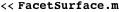The function FacetSurfaceGridData (defined in FacetSurface.m) generates the “elevation data” that defines a rough surface. This function in turn uses the built-in commands Nest and CellularAutomaton to accumulate steps of a two-dimensional, two-state, totalistic, nine-neighbor, cellular automaton evolution. The following example shows a surface defined by the two-dimensional totalistic cellular automaton rule 450 on a 21×15 grid and accumulating five steps of the evolution from the default initial conditions.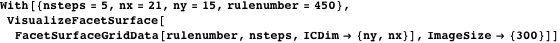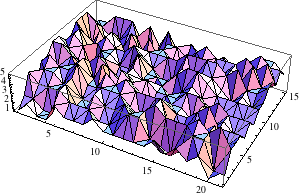Figure 1. A sample irregular surface generated with rule 450.

The FacetSurfaceGridData function uses the built-in CellularAutomaton command, which may be unfamiliar to some readers. Consequently, to explain the options and operation of the FacetSurfaceGridData function, we next consider the basics of two-dimensional cellular automaton computation.

##### Basic Idea: Two-Dimensional Cellular Automaton Accumulations

First, we deconstruct the phrase “accumulate steps of a two-dimensional, two-state, totalistic, nine-neighbor, cellular automaton evolution.”

1. “A two-dimensional cellular automaton evolution” means that a two-dimensional array of “cells” is computed (in an “evolution”) for a prescribed number of steps. Each node in this two-dimensional array is a distinct cell.
2. The “two-state” qualifier means that each cell can embody only one of exactly two states at any given step of the evolution. The CellularAutomaton function, by convention, labels these two cellular automaton states 0 and 1.
3. At each step in the evolution, each cell’s value (0 or 1) in the grid is updated, in parallel, to a new value (0 or 1), using a cell-by-cell update protocol called the “cellular automaton rule.”
4. The phrase “totalistic, nine-neighbor” specifies the type of update rule employed; here, it means that the sum of a given cell’s nine-cell neighborhood (the 3×3 block of cells centered on the evaluation cell) is looked up in a table to determine the state of the middle cell in the next step.

So, the computation of a single step of a totalistic cellular automaton evolution consists of—for each cell—summing the states of that cell’s neighboring cells and using this sum to look up the cell’s next state from a table. The look-up table is obtained from the cellular automaton rule number. Figure 1 above was generated using two-state totalistic nine-neighbor rule 450, and this is the rule’s corresponding look-up table.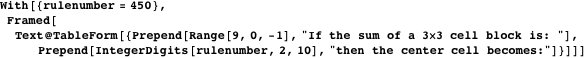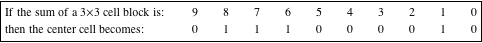Figure 2. Totalistic, nine-neighbor, 2-state cellular automaton transformation table for rule 450.

The interpretation of the above table is, for example: if all nine cells in a 3×3 block are in state 1, then their sum is 9 and the center cell would be set to 0; if all but one of these nine cells are in state 1, their sum is 8 and the center cell would be set to state 1; and so on.

The rule-decomposition convention illustrated in Figure 2 is a powerful device, because it provides both a succinct description of the look-up table and a means of enumerating all of the possible rules. For example, consider nine-neighbor, two-state, totalistic rules. This class of look-up table needs 10 entries (0, 1, …, 9) and there are 2 possibilities (0, 1) for each entry, so there are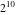possible look-up tables, and the rules for these tables are numbered 0, 1, 2, …, 1023. This convention for enumerating cellular automaton transformation rules is due to Wolfram .

Now let us visualize the underlying cellular automaton evolution that was used to generate the specific surface shown in Figure 1 above.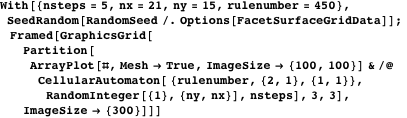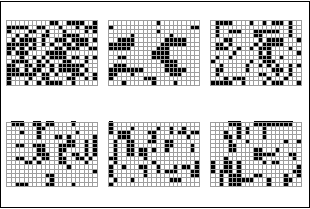Figure 3. These individual cellular automaton evolution steps are summed, cell by cell, to give the elevations for the surface depicted in Figure 1. Here, the cells in state “1” are black, cells in state “0” are white, and the evolution protocol is the totalistic nine-neighbor rule 450.

If the images in Figure 3 are “stacked” together by summing corresponding cells, the resulting array of numbers corresponds to the irregular-surface elevations used in the visualization of Figure 1. This is what is meant by “an accumulation” of a cellular automaton evolution.

One might reasonably ask if it would be possible to deduce, based on the rule number, exactly what sort of rough surface would be produced. A related question is whether one could predict the seemingly random evolution of rule 450 (shown in Figure 3) from an analytical deconstruction of rule 450’s look-up table, shown in Figure 2. Although cellular automaton evolutions are deterministic, one cannot in general predict the evolution of an arbitrary rule from a systematic analysis of the rule’s protocol. Consequently, Wolfram has argued that the only certain means of assessing the behavior of a particular rule/initial-condition combination is to run the computation and visualize the result .

##### Surface Variations

Since cellular automaton evolutions are deterministic, the random-looking surfaces produced with VisualizeFacetSurface can be reproduced identically, each time, for the same set of input parameters. Topological variations arise with changes to any of the following:

• the number of computation steps (typically, 3-20 are used in this article);
• the size or shape of the grid;
• the initial conditions;
• the cellular automaton rule (there are 1024 possibilities).

To illustrate the effect of varying these parameters, consider the four possible surfaces produced for exactly two rule numbers and two initial conditions, with all other parameters held constant. Each row in Figure 4 corresponds to a specific rule number and each column corresponds to a specific initial condition.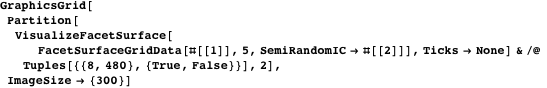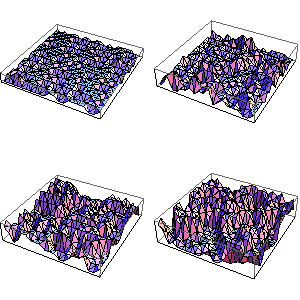Figure 4. Each column represents the same initial condition and each row the same rule number (row 1: rule 8, row 2: rule 480). All other parameters are the same for all four surfaces.

For more details about the implementation and the options for FacetSurfaceGridData, see the package FacetSurface.m. We now turn to visualizing irregular surfaces.

### Plotting Nonsmooth Data Arrays

#### Motivating Triangular-Facet Visualization

It is useful to visualize a data array as a three-dimensional surface, and normally the Mathematica function ListPlot3D is exactly the tool to use. The following example compares ListPlot3D and VisualizeFacetSurface for a regular data array.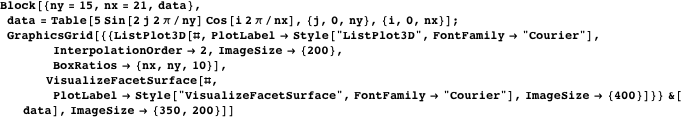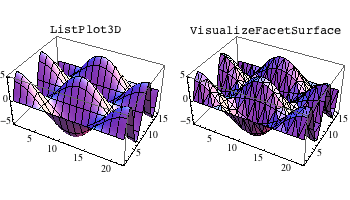Figure 5. Comparing ListPlot3D and VisualizeFacetSurface on a smooth data array.

ListPlot3D employs interpolation schemes to give a smooth surface appearance, which is a desirable treatment for regular data (although the rendering of the VisualizeFacetSurface image is considerably faster).

Let us repeat this visualization comparison with nonsmooth (random) data.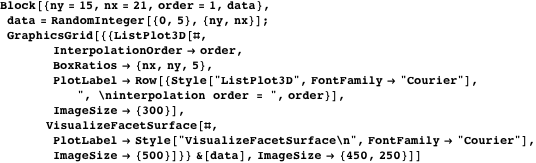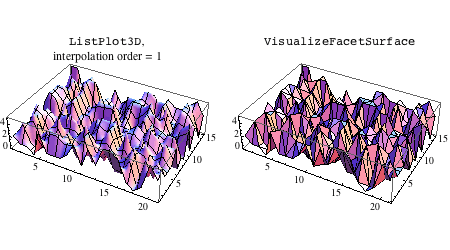Figure 6. Comparing ListPlot3D and VisualizeFacetSurface on an array of irregular data.

Figure 6 suggests that the triangular-facet visualization of nonsmooth data (VisualizeFacetSurface) has a crisper appearance. This is because ListPlot3D, even with a low-order interpolation method (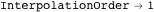), gives a visualization with cusps and curvatures that are artifacts of interpolation.

#### How VisualizeFacetSurface Works

ListPlot3D and VisualizeFacetSurface employ the same conventions for visualizing a data array (say,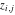) as a surface:

• the column positionis treated as the node’s horizontal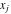displacement;
• the row positionis treated as the node’s vertical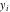displacement;
• the data array valueis interpreted as the node’s elevation.

On the other hand, VisualizeFacetSurface visualizes the data using flat triangular facets arranged in an alternating-diagonal pattern. To achieve this effect, we use the following pattern of alternating diagonals, imposed on thenodal grid, as shown in Figure 7.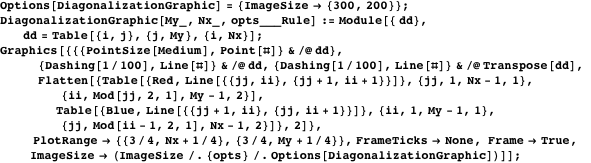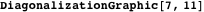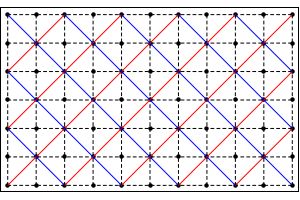Figure 7. The diagonalization convention for VisualizeFacetSurface and TriangulatedInterpolation.

This diagonalization specifies a set of triangular facets that in turn articulate thedata as if it were a continuous surface. The (interior) nodes of Figure 7 correspond to vertex points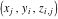that are common to either four or eight triangular facets.

In summary, the VisualizeFacetSurface function visualizes an arrayusing alternating-diagonal triangular facets. To further interpolate points, using array, in a manner consistent with this visualization, we next consider the function TriangulatedInterpolation.

#### TriangulatedInterpolation

TriangulatedInterpolation is a function for interpolating the data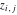at non-nodal points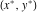in a way that is consistent with VisualizeFacetSurface.

The interpolation nodes have horizontal and vertical offsets,that are in effect (integer) array indices, with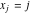and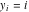. However, an arbitrary interpolation pointnormally entails noninteger quantities and thus falls between four nearest-neighbor node points, given by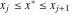and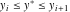.

Figure 8 illustrates the conventions used in the definition of the TriangulatedInterpolation function, including the counterclockwise numbering of the four nodes 1, 2, 3, 4 surrounding the interpolation point, beginning with node,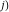, and the quantities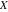and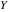, which correspond to theanddistances of the interpolation pointfrom node 1.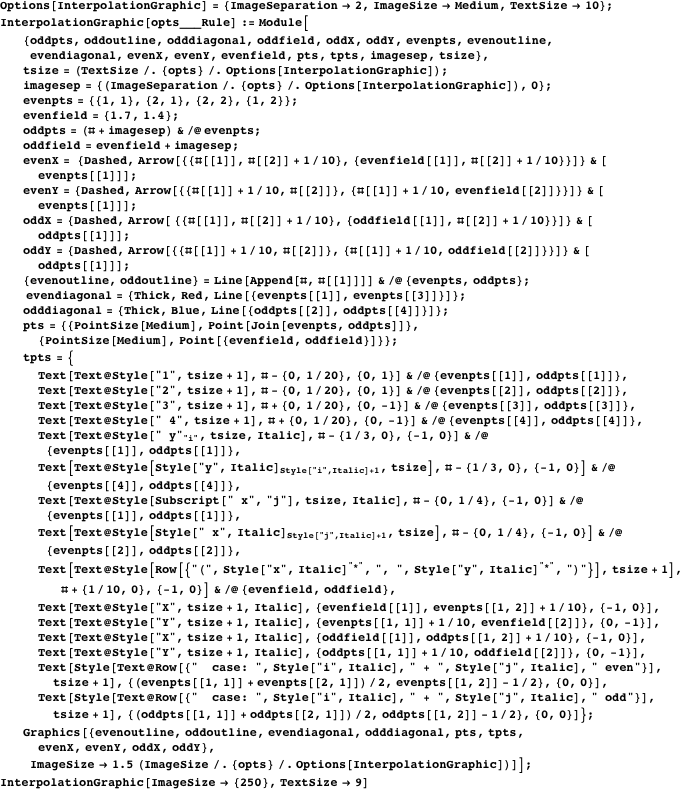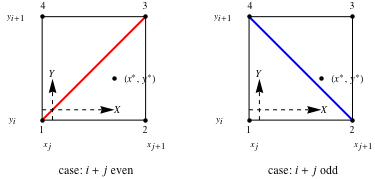Figure 8. The four possible interpolation triangles for field point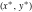.

The principal complication in alternating-diagonal triangulated-facet interpolation is identifying the appropriate interpolation triangle, because once this is determined, the interpolation is readily computed from this triangle’s three nodal vertices using basic geometry. Figure 8 illustrates that there are four different cases, depending on which diagonal is drawn and whether or not the point lies above or below the diagonal. The four cases are:

1.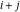even
2.below the diagonal: nodes 1, 2, and 3 are used to interpolate;above the diagonal: nodes 1, 3, and 4 are used to interpolate;
3.odd
4.below the diagonal: nodes 1, 2, and 4 are used to interpolate;above the diagonal: nodes 2, 3, and 4 are used to interpolate.

These are the core ideas implemented in TriangulatedInterpolation. To illustrate the function call, in Figure 9 a circle is drawn on the random-data surface visualized in Figure 6.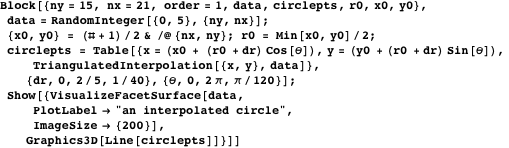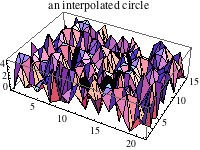Figure 9. Drawing a circle on a rough surface using the TriangulatedInterpolation function.

### Summary

The first is a new method to simulate irregular surfaces that uses totalistic cellular automata computations to generate the surface-elevation data. The method is compactly implemented with built-in Mathematica functions. By varying the computation parameters, singly or in combination, one may achieve a variety of effects. Surfaces generated by cellular automata are less homogeneous in character compared to surfaces simulated by either statistical or fractal methods.

The second capability relates to the visualization and interpolation of irregular-data arrays, using an alternating-diagonal triangular-facet representation. This basic visualization scheme provides a crisp articulation of rough surfaces. A corresponding interpolation procedure provides a consistent treatment of non-nodal field points.

### Acknowledgments

These algorithms were conceived during the author’s participation at the NKS 2008 Summer School in Burlington, Vermont, sponsored by Wolfram Research. The author is grateful for the experiences she gained there.

### References

  J. A. Greenwood and J. B. P. Williamson, “Contact of Nominally Flat Surfaces,” Proceedings of the Royal Society A, 295, 1966 pp. 300-319. doi:10.1098/rspa.1966.0242.  N. Patir, “A Numerical Procedure for Random Generation of Rough Surfaces,” Wear, 47(2), 1978 pp. 263-277. doi:10.1016/0043-1648(78)90157-6.  M. S. Hong and K. F. Ehmann, “Three-Dimensional Surface Characterization by Two-Dimensional Autoregressive Models,” Journal of Tribology, 117(3), 1995 pp. 385-393. doi:10.1115/1.2831263.  H. Sofuoglu and A. Ozer, “Thermomechanical Analysis of Elastoplastic Medium in Sliding Contact with Fractal Surface,” Tribology International, 41(8), 2008 pp. 783-796. doi:10.1016/j.triboint.2008.01.010.  D. Goerke and K. Wilner, “Normal Contact of Fractal Surfaces—Experimental and Numerical Investigations,” Wear, 264(7–8), 2008 pp. 589-598. doi:10.1016/j.wear.2007.05.004.  Y. H. Jang, “Distribution of Three-Dimensional Islands from Two-Dimensional Line Segment Length Distribution,” Wear, 257(1–2), 2004 pp. 131-137. doi:10.1016/j.wear.2003.10.020.  M. Zou, B. Yu, Y. Feng, and X. Peng, “A Monte Carlo Method for Simulating Fractal Surfaces,” Physica A, 386(1), 2007 pp. 176-186. doi:10.1016/j.physa.2007.07.058.  D. Blackmore and J. G. Zhou, “A General Fractal Distribution Function for Rough Surface Profiles,” SIAM Journal of Applied Mathematics, 56(6), 1996 pp. 1694-1719. doi:10.1137/S0036139995283122.  V. Bakolas, “Numerical Generation of Arbitrarily Oriented Non-Gaussian Three-Dimensional Rough Surfaces,” Wear, 254(5–6), 2003 pp. 546-554. doi:10.1016/S0043-1648(03)00133-9.  S. Wolfram, “Universality and Complexity in Cellular Automata,” Physica D, 10, 1984 pp. 1-35. www.stephenwolfram.com/publications/articles/ca/84-universality.  S. Wolfram, A New Kind of Science, Champaign, Illinois: Wolfram Media, Inc., 2002. V. J. Peridier, “FacetSurface Representation,” The Mathematica Journal, 2011. dx.doi.org/doi:10.3888/tmj.13-11.